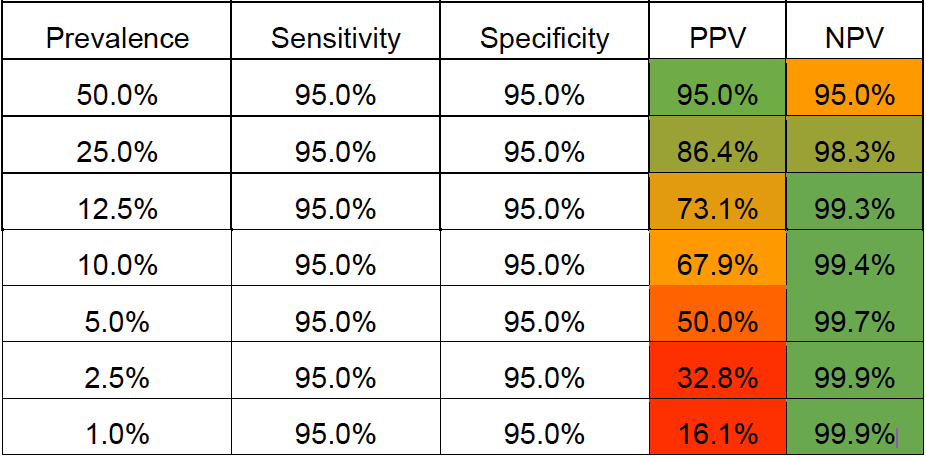# How to Evaluate AI Accuracy in RadiologySince medical imaging AI is often used as a diagnostic-aid tool, algorithms are evaluated on accuracy the same way as most other medical tests. From a technical perspective, the sensitivity and specificity of a solution — the true positive and true negative rate, respectively — are helpful when determining the approximate accuracy of an AI solution. But for a more real-world, user experience-related metric, we can look at the positive and negative predictive values to see the probability of positive or negative alerts being true.

## Sensitivity and Specificity

The sensitivity of a tool, or the true positive rate, is the most intuitive way of measuring accuracy. This is simply the percentage of positive results that are correct. For example, in an academic study on our pulmonary embolism (PE) solution, researchers discerned a sensitivity of 92.7%, meaning the AI correctly identified 215 positive cases of PE out of the actual 232 positive cases.

On the other hand, the specificity of the tool, or the true negative rate, is the opposite of sensitivity. Specificity is the percentage of negative cases that the tool correctly identifies as negative. In the same academic study of our PE solution mentioned above, the authors determined a specificity of 95.5%. This means the AI correctly identified 1178 negative cases out of the actual 1233 negative cases.

## Positive Predictive Value — Probability That a Positive Result is Accurate

The positive predictive value of a tool, or PPV, is the probability that a positive result is actually positive. It’s easiest to think of PPV as the “spam” metric, or the chance of seeing an irrelevant alert. The lower the PPV, the higher chance that a positive notification can be disregarded as false.

For example, let’s say an AI analyzes 1000 CT images of patients’ spines, searching for C-spine fractures. There are 100 cases of actual fractures, and the AI spots 95 of them. It also incorrectly flags another 90 cases as fractures, when there are actually none. To calculate the PPV, we take the number of true positives (95) and divide that by all 185 positive calls (95 true positives + 90 false positives), giving us a PPV of 51%. A radiologist using this AI tool should probably give each positive alert a good look over, since the chance of each one being accurate is only around 50%.

## Negative Predictive Value — Probability That a Negative Result is Accurate

The negative predictive value of a tool, or NPV, is the probability that a negative result is actually negative. NPV can be thought of as the “peace of mind” metric, or how sure you can be if the AI says the case is negative. The higher the NPV,  the more confident you can be in the tool’s negative calls.

It is common to see very high NPVs in most diagnostic tools since most patients don’t actually have the condition being tested for. Generally speaking, most AI solutions will have NPV values of 97% and higher. Going back to the C-spine example from the PPV section, even if the sensitivity and specificity were only 80% each, you would still see an NPV of 97.5%. This is because all well-developed algorithms always err on the side of caution, marking ambiguous cases as positive to prevent potentially dangerous false negatives.

## Prevalence

As briefly mentioned above, disease or abnormality prevalence is relatively low in a typical setting, around 2%-15%. Depending on the exact numbers, an AI could still be exceptional if it had a PPV in the 50%-70% range. For rare abnormalities, a PPV as low as 20% could still represent excellent performance! NPV should, however, be high. You should look for an NPV of 95% or higher for reliable AI systems.

It’s important to note that the sensitivity + specificity balance for a specific disease prevalence can ultimately impact the user experience, i.e., a low PPV with many irrelevant alerts can ultimately result in a high level of alert fatigue.

Here’s an example of how PPV and NPV may vary for the exact same algorithm sensitivity and specificity as disease prevalence varies.In short, positive predictive value (PPV) is the metric that users are most familiar with – it’s the most ‘real-world’ statistic. PPV is highly dependent on the prevalence of a pathology – as we saw in the table above, algorithms searching for low-prevalence conditions have significantly lower PPVs than higher prevalence conditions. Sensitivity and specificity are currently the standard way of evaluating algorithms from a technical perspective, and you’ll usually see these in academic studies of medical imaging AI. In the near future, we may even see a whole new metric emerge that enables a more precise evaluation of radiology AI algorithms.

## Latest Posts

##### Subscribe to our Blog